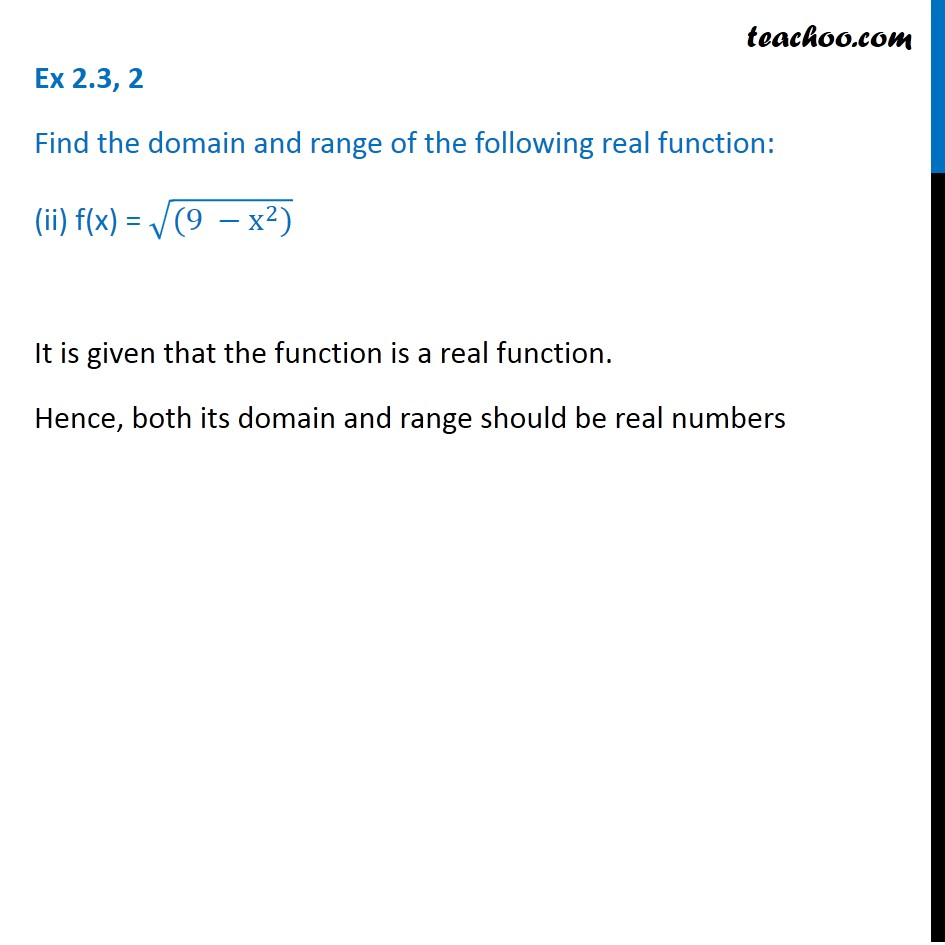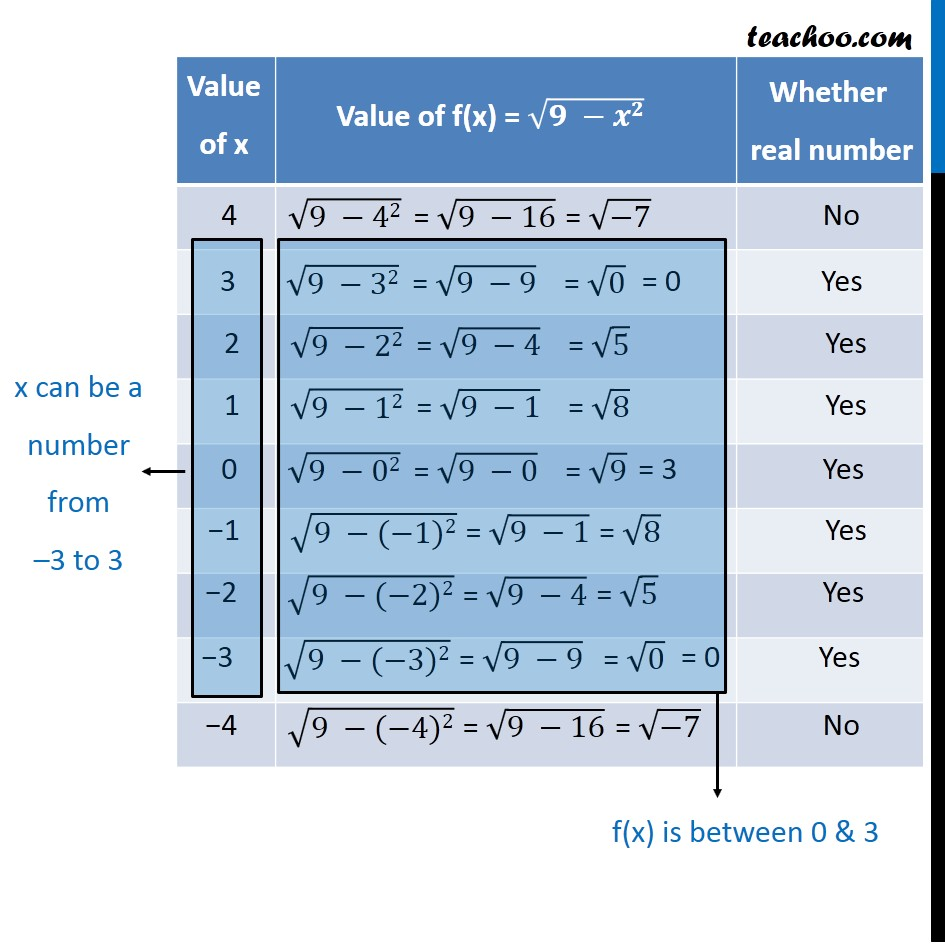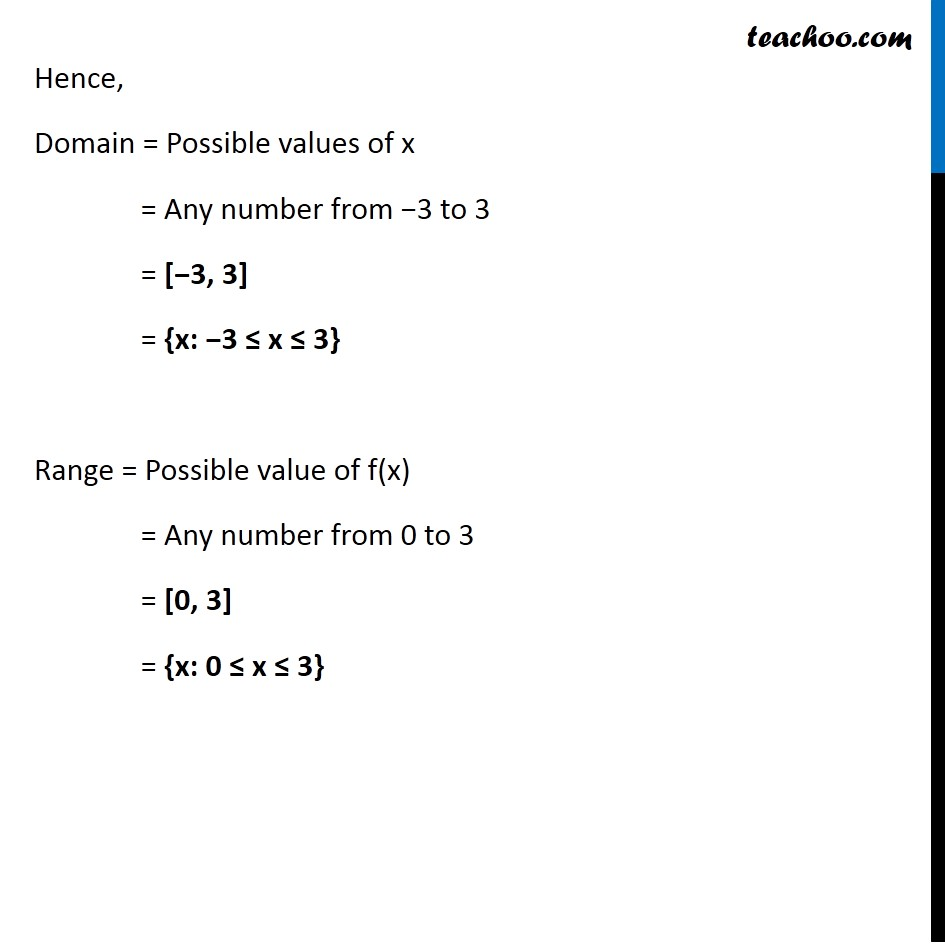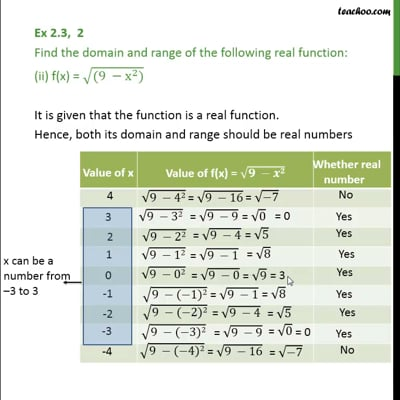Ex 2.3

Chapter 2 Class 11 Relations and Functions (Term 1)
Serial order wiseThis video is only available for Teachoo black users

### Transcript

Ex 2.3, 2 Find the domain and range of the following real function: (ii) f(x) = √((9 −x^2)) It is given that the function is a real function. Hence, both its domain and range should be real numbers x can be a number from –3 to 3 f(x) is between 0 & 3 Hence, Domain = Possible values of x = Any number from −3 to 3 = [−3, 3] = {x: −3 ≤ x ≤ 3} Range = Possible value of f(x) = Any number from 0 to 3 = [0, 3] = {x: 0 ≤ x ≤ 3}